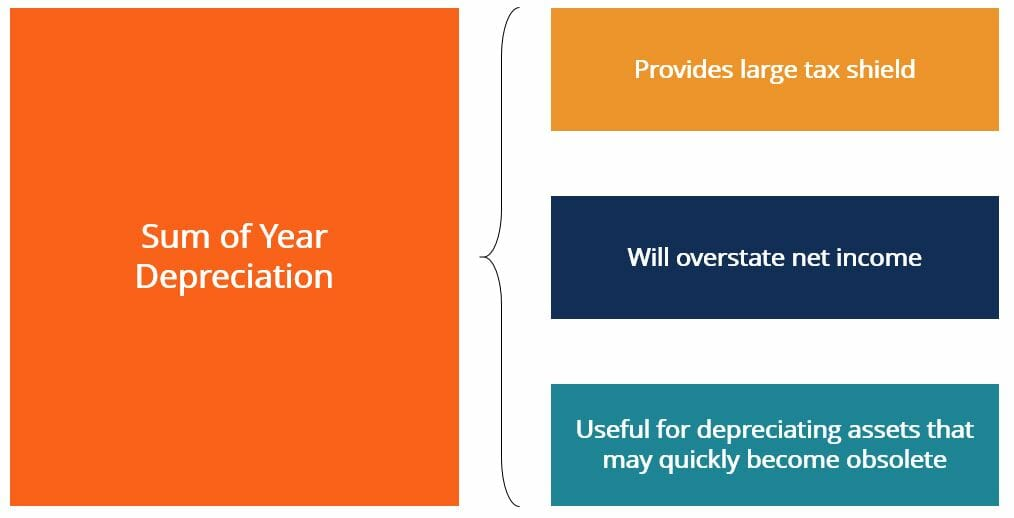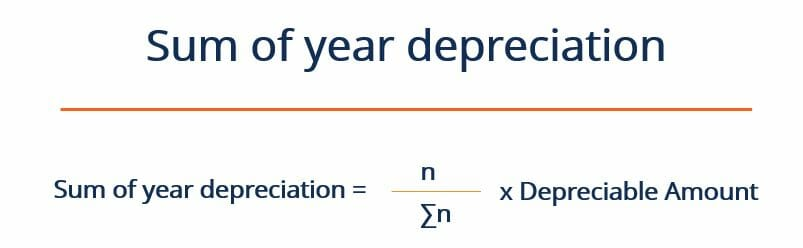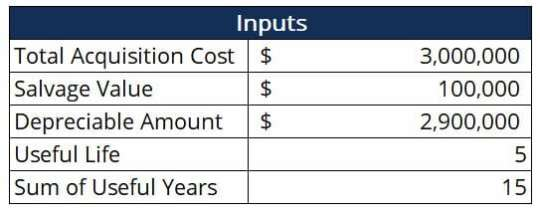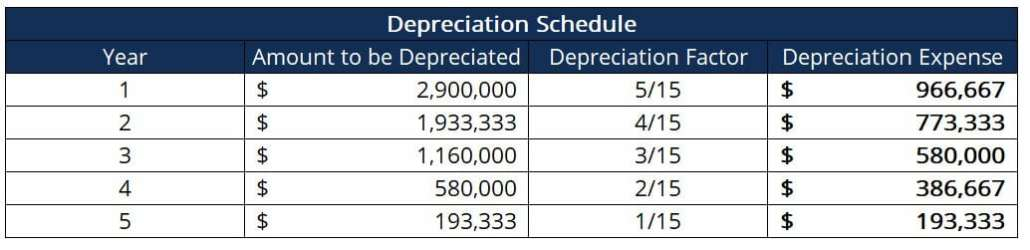# Sum of Year Depreciation

An alternative accelerated depreciation method

## What is Sum of Year Depreciation?

Sum of Year Depreciation (SYD) is a method of accelerated depreciation. Similarly to the double declining balance method, sum of year depreciation aims to depreciate a company’s assets at an accelerated rate. Companies may choose to do this as this practice will result in a bigger depreciation tax shield in the first few years of the depreciation.Organizations that face difficult tax environments may choose to depreciate their assets in an accelerated way in order to realize larger tax savings and benefit from the overstated net income figure shown on the company’s financial statements.

The sum of year method of depreciation is also popular with firms that are looking to write off equipment that has a high chance of becoming obsolete before the salvage value is reached. For example, a company may choose this method to depreciate assets such as computers, which may become obsolete very quickly given the rate of technological advancements in the world today.

### How does sum of year depreciation work?

The sum of year depreciation method works by depreciating the asset’s depreciable amount by a depreciation factor unique to each year. The depreciable amount is equal to the asset’s total acquisition cost less the asset’s salvage value. The total acquisition cost refers to the total capital expenditure that the company had to undertake in order to gain possession of said assets.

The total cost would include the purchase price of the asset, any shipping costs associated with moving the asset to the company, and any installation costs. The depreciation factor is the useful life of the asset (in years) divided by the sum of all the useful years. The formula below summarizes the process:Where:

n – Useful life of the asset (ex. 4 years)

∑n – Sum of years (ex. 4 years: 1+2+3+4 = 10)

Depreciable amount – (Total Acquisition Cost – Salvage Value)

### Sum of year depreciation example

Consider coffee company Mega Coffee, which is ready to expand into its new office headquarters. The company is considering investing in the latest available computers in order to make sure that its business runs smoothly. The material cost of all the computers is \$2,500,000. However, Mega Coffee has to pay \$100,000 in shipping costs in order to move this massive order of computers across the country in due time. In addition to this, Mega Coffee is faced with a \$100,000 installation charge to ensure that its computers are installed correctly and function at full capacity.

Mega Coffee believes that at the end of the computers’ 5-year useful life, they will be worth \$200,000. The company decides to depreciate these assets using the SYD method as it faces a fairly harsh tax environment, and because there is a chance that the computers becoming obsolete before their useful life is up. Create a depreciation schedule to model how these assets can be depreciated.

##### Solution

The first step is to identify the main inputs needed for this calculation. These are summarized in the table below:Where:

Total acquisition cost – \$2,500,000 + \$100,000 + \$400,000 = \$3,000,000

Depreciable Amount – \$3,000,000 – \$100,000 = \$2,900,000

Sum of useful years – 1+2+3+4+5 = 15

Once we have established the relevant parameters, we can calculate the depreciation expense for each year as follows:Notice how the depreciation expense in year 1 (\$966,667) is about five times greater than the depreciation expense in year 5 (\$193,333). Thus, if the computers were to become obsolete after year 3, a huge part of their value would have already been depreciated. This means that the company would have already realized most of the tax benefits associated with depreciation, and thus would be more inclined to invest in more advanced technology.

CFI offers the Financial Modeling & Valuation Analyst (FMVA)™ certification program for those looking to take their careers to the next level. To learn more about related topics, check out the following resources:

• Depreciation Expense
• Accumulated Depreciation
• IFRS Standards
• Cash Flow Statement